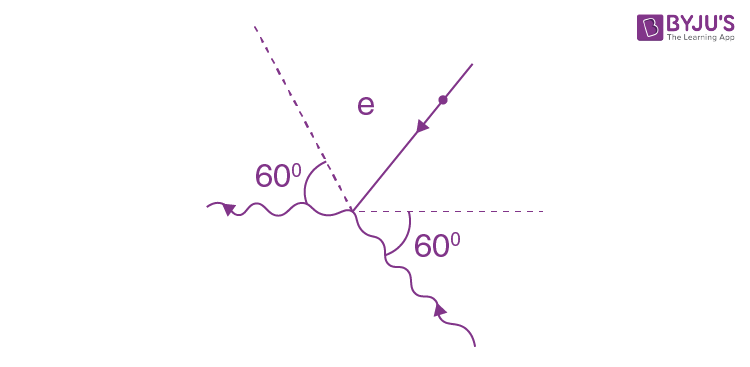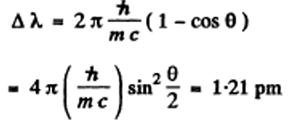# I.E. Irodov Solutions on Thermal Radiation

Thermal Radiation is the transfer of heat by the means of the electromagnetic radiation generated by the thermal motion of particles in matter. Chapter 5.7 - Thermal Radiation,(Quantum Nature of Light) solutions of I.E. Irodov book are provided on this page to help students understand the concept in a better way. I.E. Irodov chapter 5.7 - Thermal Radiation problems are solved by subject experts in a detailed way. Students can refer to the solutions and develop gain better efficiency in tackling complex and difficult questions that students might come across while learning the different concepts.

In order to make studying easier, the I.E. Irodov Solutions provided here offers the right knowledge that will further help them be proficient with the terminologies and concepts. In essences, students will be able to solve problems effectively and perform well in exams like IIT JEE amongst others.

### I.E. Irodov Solutions on Thermal Radiation

1: The temperature of one of the two heated black bodies is T1 = 2500 K. Find the temperature of the other body if the wavelength corresponding to its maximum emissive capacity exceeds by Δλ = 0.50 gm the wavelength corresponding to the maximum emissive capacity of the first black body.

Solution:

1. For the first black body

m)1 = b/T1

Then, (λm)2 = b/T1 + ∆λ = b/T2

Hence, T_2 = \frac{b}{\frac{b}{T_1} + \Delta \lambda}=\frac{bT_1}{b+T_1 \Delta \lambda}=1.747 kK

2: The radiosity of a black body is Me = 3.0 W/cm2. Find the wavelength corresponding to the maximum emissive capacity of that body.

Solution:

1. From the radiosity we get the temperature of the black body. It is

T = (Me/ σ)1/4 = [(3x104)/(5.67x10-8)]1/4 = 852.9 K

Hence the wavelength corresponding to the maximum emissive capacity of the body is

b/T = 0.29/852.9 cm = 3.4 μm

3: The spectral composition of solar radiation is much the same as that of a black body whose maximum emission corresponds to the wavelength 0.48μm. Find the mass lost by the Sun every second due to radiation. Evaluate the time interval during which the mass of the Sun diminishes by 1 per cent.

Solution:

1. The black body temperature of the sun maybe taken as

T θ =0.29/[0.48x10-4]=6042 K

M = 5.67 x 10-8 (6042)4 = 0.7555 x 108 W/m2

Energy lost by sun is

4 π(6.95)2 x 1016 x 0.7555 x 108 = 4.5855 x 1026 watt

This corresponds to a mass loss of

[4.5855x1026]/[9x1016] Kg/sec = 5.1 x 109 kg/sec

The sun loses 1 % of its mass in

[1.97x1030x10-2]/[5.1x109] sec = 1.22 x 1011 years.

4: Find the temperature of totally ionized hydrogen plasma of density ρ = 0.10 g/cm3 at which the thermal radiation pressure is equal to the gas kinetic pressure of the particles of plasma. Take into account that the thermal radiation pressure ρ = u/3, where u is the space density of radiation energy, and at high temperatures all substances obey the equation of state of an ideal gas.

Solution:

1. For an ideal gas p = n k T where n = number density of the particles and k = R/NA is Boltzman constant. In a fully ionized hydrogen plasma, both H ions (protons) and electrons contribute to pressure but since the mass of electrons is quite small only protons contribute to mass density.

Thus, n = 2 ρ/mH and p = 2 ρRT/NAmH

Where mp = mH is the proton or hydrogen mass.

Equating this to thermal radiation pressure

2 ρRT/NAmH = u/3 = Me/3 x 4/c = 4σT4/3c

Then, T3 = 3cρR/2σNAmH = 3cρR/σM

Where M = 2NAmH = molecular weight of hydrogen = 2 x 10-3 kg

Thus, T = (3cρR/σM)1/3 = 1.89 x 107 k (approx.)

5: A copper ball of diameter d = 1.2 cm was placed in an evacuated vessel whose walls are kept at the absolute zero temperature. The initial temperature of the ball is To = 300 K. Assuming the surface of the ball to be absolutely black, find how soon its temperature decreases η = 2.0 times.

Solution:

1. In time dt after the instant t when the temperature of the ball is T, it loses πd2σT4 dt

Joules of energy, its temperature falls by - dT and πd2σT4 dt = (-π/6)d3ρC dT

Where ρ = density of copper, C = its specific heat

So, dt = [-Cρd/6σ] dT/T4

t_o =\frac{C \rho d}{6 \sigma}\int_{T_0}^{T_o/\eta} -\frac{dT}{T^4} =\frac{C \rho d}{18 \sigma T_0^3}(\eta^3-1)

=2.94 hours

6: There are two cavities (Fig. 5.39) with small holes of equal diameters d = 1.0 cm and perfectly reflecting outer surfaces. The distance between the holes is l = 10 cm. A constant temperature T1 = 1700 K is maintained in cavity 1. Calculate the steady-state temperature inside cavity 2. Instruction. Take into account that a black body radiation obeys the cosine emission law.Solution:

1. Taking account of cosine low of emission we write for the energy radiated per second by the hole in cavity # 1 as

dI(Ω) = A cos θ dΩ

where A is an constant, dΩ is an element of solid angle around some direction defined by the symbol Ω . Integrating over the whole forward hemisphere we get

I = A \int_0^{\pi/2}cos \theta 2 \pi sin \theta d\theta =\pi A

We find A by equating this to the quantity σT14 (πd2/4) σ is stefan-Boltzman constant and d is the diameter of the hole.

Then, A = (1/4)σd2T14

Now energy reaching 2 from 1 is (cosθ = 1)

(1/4)σd2T14 . ∆ Ω

where ∆ Ω = (πd2/4)/l2 is the solid angle subtended by the hole of 2 at 1. {We are assuming d < <l so ∆ Ω = (area of hole)/(distance)2

= (1/4)σπd2T24

Which is energy emitted by 2, thus (1/4)σd2T14 [πd2/4l2] = (1/4)σπd2T24

T2 = T1√(d/2l)

Put T2 = 0.380kK = 380 K

7: A cavity of volume V = 1.0 l is filled with thermal radiation at a temperature T = 1000 K. Find: (a) the heat capacity Cv ;

(b) the entropy S of that radiation.

Solution:

1. (a) The total internal energy of the cavity is

U = (4σ/c)T4 V

Hence, Cv = (\frac{\partial U}{\partial T})_V

= (16σ/c)T3 V

On substituting the values, we have

= 3.024 nJ/K

(b) From first law, TdS = dU + pdV

= V dU + Udv + (u/3)dV (here p = U/3)

= VdU + 4U/3 dV

= (16σ/C)VT3 dT + (16σ/3C)T4 dV

So, dS = (16σ/C)VT2 dT + (16σ/3C)T3 dV

= d(16σ/3C)VT3)

Hence, S = (16σ/3C)VT3 = (1/2) Cv = 1.008 nJ/K

8: Using Planck's formula, derive the approximate expressions for the space spectral density uω of radiation

(a) in the range where hω << kT (Rayleigh-Jeans formula);

(b) in the range where Nω >> kT (Wien's formula).

Solution:

1. From Planck’s formula

u_\omega=\frac{h \omega^3}{\pi^2 c^3}\frac{1}{e^{h\omega/kT}-1}

(a) In a range h ω << kT (long wavelength or high temperature).

u_\omega \rightarrow \frac{h \omega^3}{\pi^2 c^3}\frac{1}{h \omega/KT}

u_\omega = \frac{ \omega^2}{\pi^2 c^3}kT

Using ex = 1+x, for small x.

(b) In the range hω >>> T (high frequency or low temperature)H

hω/kT >> 1 so ehω/kT >> 1

and u_\omega = \frac{h \omega^3}{\pi^2 c^3}e^{-h\omega/kT}

*where h=plank constant

9: Transform Planck's formula for space spectral density uω of radiation from the variable ω to the variables v (linear frequency) and λ (wavelength).

Solution:

1. we know, u_\omega d\omega=\tilde{u}_v dV where ω = 2πv, then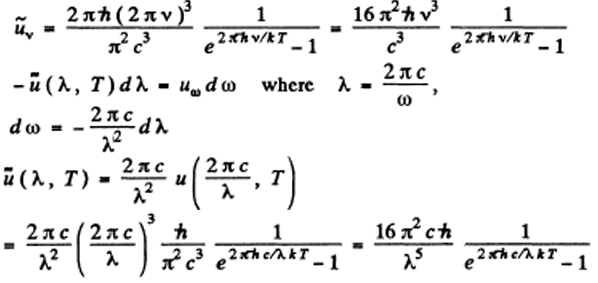10: Using Planck's formula, find the power radiated by a unit area of a black body within a narrow wavelength interval Δλ = 1.0 nm close to the maximum of spectral radiation density at a temperature T = 3000 K of the body.

Solution:

1. We write the required power in terms of the radiosity by considering only the energy radiated in the given range. We know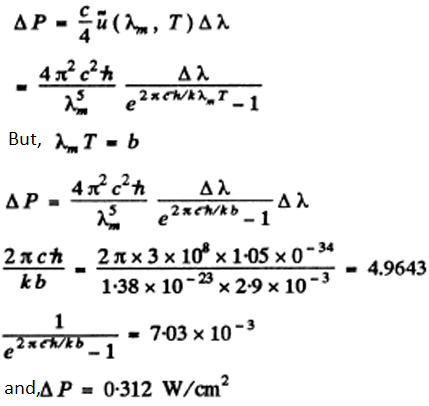11: From the standpoint of the corpuscular theory demonstrate that the momentum transferred by a beam of parallel light rays per unit time does not depend on its spectral composition but depends only on the energy flux Θe.

Solution:

1. The statement made in the question is not always correct. However it is correct in certain cases, for example, when light is incident on a perfect reflector or perfect absorber.

Consider the former case. If light is incident at an angle θ and reflected at the angle θ, then momentum by each photon is (2hv/c) cos θ.

If there are n(v) dv photons in frequency interval (v, v + dv), then total momentum transferred is

\int_0^\infty 2n(v) \frac{hv}{c} cos \theta dv

= [2ϕe/c] cos θ

12: A laser emits a light pulse of duration ζ = 0.13 ms and energy E = 10 J. Find the mean pressure exerted by such a light pulse when it is focussed into a spot of diameter d = 10 pm on a surface perpendicular to the beam and possessing a reflection coefficient ρ = 0.50.

Solution:

1. The mean pressure <p> is related to the force F, exerted by the beam by <p> = x(πd2/4) = F

The force F equals momentum transfered per second. This is (assuming that photons, not reflected, are absorbed).

2 \rho \frac{E}{c \tau}+(1-\rho)\frac{E}{c \tau} = (1+\tau)\frac{E}{c \tau}

The first term is the momentum transfered on reflection; the second on absorption

<p> = [4(1+ρ)E]/πd2c𝜏

on substituting the values we have <p> = 48.3 atm

13: A plane light wave of intensity I = 0.70 W/cm2 illuminates a sphere with ideal mirror surface. The radius of the sphere is R = 5.0 cm. From the standpoint of the corpuscular theory find the force that light exerts on the sphere.

Solution:

1. We consider a strip defined by the angular range (θ , θ+dθ).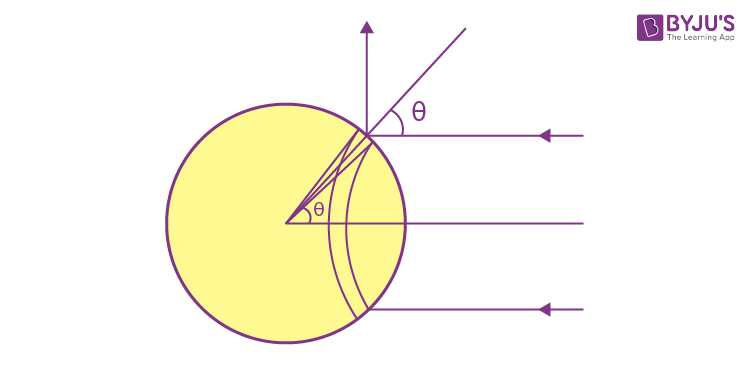We know, the normal pressure exerted on this strip is (2I/c) cos2 θ

This pressure gives rise to a force whose resultant, by symmetry is in the direction of the incident light. Thus,

F = \frac{2I}{c} \int_0^{\pi/2}cos^2 \theta cos\theta. 2\pi R^2 sin\theta d\theta = \pi R^2 \frac{I}{c}

Putting in the value, we get F = 0.183 μN

14: A voltage applied to an X-ray tube being increased η = 1.5 times, the short-wave limit of an X-ray continuous spectrum shifts by Δλ = 26 pm. Find the initial voltage applied to the tube.

Solution:

1. The general formula,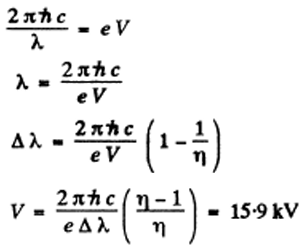15: A narrow X-ray beam falls on a NaC1 single crystal. The least angle of incidence at which the mirror reflection from the system of crystallographic planes is still observed is equal to α = 4.1°. The interplanar distance is d = 0.28 nm. How high is the voltage applied to the X-ray tube?

Solution:

1. we know, 2 πhc/ λ = eV

From Bragg’s law, 2d sin α = k λ = λ

Since k = 1 , at α = smallest value

So, V = πhc/ ed sin α = 31 kV (approx.)

16: Find the maximum kinetic energy of photoelectrons liberated from the surface of lithium by electromagnetic radiation whose electric component varies with time as E = a (1 + cos ωt) cos ω0t, where a is a constant, ω = 6.0. x 1014 s-1 and ωo = 3.60.1015 s-1.

Solution:

1. Given, E = a (1 + cos ωt) cos ω0t,

= a cos ω0t +(a/2)[cos(ω0 - ω)t + cos(ω0 + ω)t]

It is obvious that light has three frequencies and the maximum K.E. of photo electrons ejected is h(ω0 + ω) - ALi

Where ALi = 2.39 eV .

Substituting we get, 0.37 eV

17: A photoelectric current emerging in the circuit of a vacuum photocell when its zinc electrode is subjected to electromagnetic radiation of wavelength 262 nm is cancelled if an external decelerating voltage 1.5 V is applied. Find the magnitude and polarity of the outer contact potential difference of the given photocell.

Solution:

1. The maximum K.E. of the photoelectrons emitted by the Zn cathode is

Emax = [2 πhc/ λ] - AZn

On calculating this comes out to be 0.993 eV ≈ 1.0 eV Since an external decelerating voltage of 1.5 V is required to cancel this current, we infer that a contact potential difference of 1.5 - 1.0 = 0.5 V exists in the circuit whose polarity is opposite of the decelerating voltage.

18: Compose the expression for a quantity whose dimension is length, using velocity of light c, mass of a particle m, and Planck's constant h. What is that quantity?

Solution:

1. The unit of h is Joule-sec. Since mc2 is the rest mass energy, h/mc2 as dimension of time and multiplying by c we get a quantity λc = h/mc

whose dimension is length. This quantity is called reduced Compton wavelength. (The name Compton wavelength is traditionally reserved for m=2 πh/mc )

19: Explain the following features of Compton scattering of light by matter: (a) the increase in wavelength Δλ, is independent of the nature of the scattering substance; (b) the intensity of the displaced component of scattered light grows with the increasing angle of scattering and with the diminishing atomic number of the substance; (c) the presence of a non-displaced component in the scattered radiation.

Solution:

1. (a) Compton scattering is the scattering of light by free electrons. (The free electrons are the electrons whose binding is much smaller than the typical energy transfer to the electrons). For this reason the increase in wavelength Δλ is independent of the nature of the scattered substance.

(b) This is because the effective number of free electrons increases in both cases. With increasing ang le of scattering, the energy transferred to electrons increases. With diminishing atomic number of the substance, the binding energy of the electrons decreases.

(c) The presence of a non-displaced component in the scattered radiation is due to scattering from strong bound (inner) electrons as well as nuclei. For scattering by these the atom essentially recoils as a whole and there is very little energy transfer.

20: Having collided with a relativistic electron, a photon is deflected through an angle θ = 60° while the electron stops. Find the Compton displacement of the wavelength of the scattered photon.

Solution:

1. This is the inverse of usual Compton scattering.

When we write down the energy-momentum conservation equation for this process we find that they are the same for the inverse process as they arc for the usual process.

If follows that the formula for Compton shift is applicable except that the energy (frequency) of the photon is ^ increased on scattering and the wavelength is shifted downward. With this understanding, we have